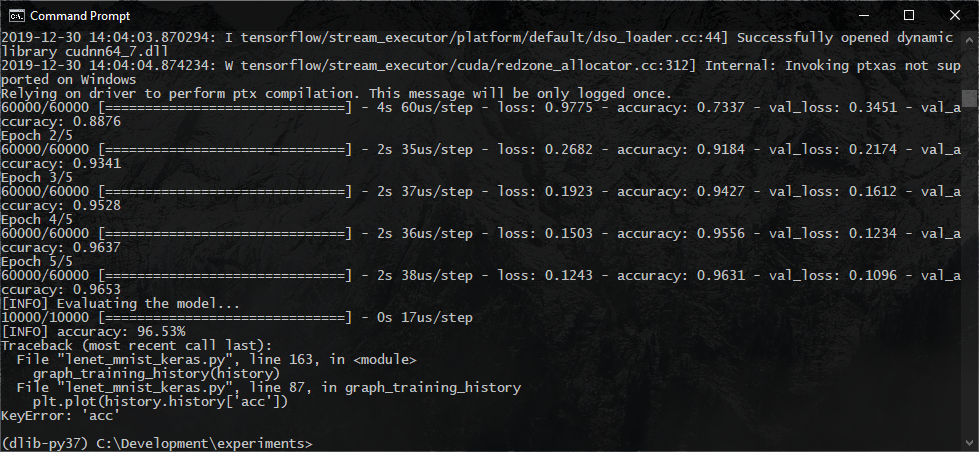# Fixing the KeyError: ‘acc’ and KeyError: ‘val_acc’ Errors in Keras 2.3.x or Newer

Original article was published by Thimira Amaratunga on Deep Learning on Medium# Fixing the KeyError: ‘acc’ and KeyError: ‘val_acc’ Errors in Keras 2.3.x or Newer

## If you’re getting errors such as KeyError: ‘acc’ or KeyError: ‘val_acc’ in your Keras code, it may be due to a recent change in Keras 2.3.x.

In Keras 2.3.0, how the matrices are reported was changed to match the exact name it was specified with. If you are using older code or older code examples, then you might run into errors. Here is how to fix them.

Have you been using the ‘History’ object returned by the fit() functions of Keras to graph or visualize the training history of your models? And have you been getting a ‘KeyError’ type error such as the following since recent Keras upgrade and wondering why?

`Traceback (most recent call last):  File "lenet_mnist_keras.py", line 163, in <module>    graph_training_history(history)  File "lenet_mnist_keras.py", line 87, in graph_training_history    plt.plot(history.history['acc'])KeyError: 'acc'`
`Traceback (most recent call last):  File "lenet_mnist_keras.py", line 163, in <module>    graph_training_history(history)  File "lenet_mnist_keras.py", line 88, in graph_training_history    plt.plot(history.history['val_acc'])KeyError: 'val_acc'`

Well, this is due to a breaking change introduced in Keras release 2.3.0.

According to the 2.3.0 Release Notes:

“Metrics and losses are now reported under the exact name specified by the user (e.g. if you pass metrics=[‘acc’], your metric will be reported under the string “acc”, not “accuracy”, and inversely metrics=[‘accuracy’] will be reported under the string “accuracy”.”

You can read the official release notes here: https://github.com/keras-team/keras/releases/tag/2.3.0

What this means is that if you specify metrics=[“accuracy”] in the model.compile(), then the history object will have the keys as ‘accuracy’ and ‘val_accuracy’. While if you specify it as metrics=[“acc”] then they will be reported with the keys ‘acc’ and ‘val_acc’.

Therefore, to fix the error, you should just use one standard across your code.

You can either use ‘acc’,

`...model.compile(loss="categorical_crossentropy",              optimizer=opt, metrics=["acc"])...plt.figure(1)# summarize history for accuracyplt.subplot(211)plt.plot(history.history['acc'])plt.plot(history.history['val_acc'])plt.title('Model Accuracy')plt.ylabel('Accuracy')plt.xlabel('Epoch')plt.legend(['Training', 'Validation'], loc='lower right')# summarize history for lossplt.subplot(212)plt.plot(history.history['loss'])plt.plot(history.history['val_loss'])plt.title('Model Loss')plt.ylabel('Loss')plt.xlabel('Epoch')plt.legend(['Training', 'Validation'], loc='upper right')plt.tight_layout()plt.show()`

Or, use ‘accuracy’.

`...model.compile(loss="categorical_crossentropy",              optimizer=opt, metrics=["accuracy"])...plt.figure(1)# summarize history for accuracyplt.subplot(211)plt.plot(history.history['accuracy'])plt.plot(history.history['val_accuracy'])plt.title('Model Accuracy')plt.ylabel('Accuracy')plt.xlabel('Epoch')plt.legend(['Training', 'Validation'], loc='lower right')# summarize history for lossplt.subplot(212)plt.plot(history.history['loss'])plt.plot(history.history['val_loss'])plt.title('Model Loss')plt.ylabel('Loss')plt.xlabel('Epoch')plt.legend(['Training', 'Validation'], loc='upper right')plt.tight_layout()plt.show()`

Just remember to use the same key in both in the metrics=[…] and where you access the keys from the history object.

Note: I first published this article on my blog — Codes of Interest — back in January 2020. But I see that many of you are still facing this issue. So I’m re-publishing it here in hopes of assisting many in fixing their code.